# Inferring Somatic Signatures from Single Nucleotide Variant Calls

Julian Gehring, EMBL Heidelberg

## 1 Motivation: The Concept Behind Mutational Signatures

Recent publications introduced the concept of identifying mutational signatures from cancer sequencing studies and linked them to potential mutation generation processes [11,2,3]. Conceptually, this relates somatically occurring single nucleotide variants (SNVs) to the surrounding sequence which will be referred to as mutational or somatic motifs in the following. Based on the frequency of the motifs occurring in multiple samples, these can be decomposed mathematically into so called mutational signatures. In case of the investigation of tumors, the term somatic signatures will be used here to distinguish them from germline mutations and their generating processes.

The SomaticSignatures package provides an efficient and user-friendly implementation for the extraction of somatic motifs based on a list of somatically mutated genomic sites and the estimation of somatic signatures with different matrix decomposition algorithms. Methodologically, this is based on the work of Nik-Zainal and colleagues . If you use SomaticSignatures in your research, please cite it as:

Gehring, Julian S., Bernd Fischer, Michael Lawrence, and Wolfgang Huber.
SomaticSignatures: Inferring Mutational Signatures from Single Nucleotide Variants.
Bioinformatics, 2015, btv408. http://dx.doi.org/10.1093/bioinformatics/btv408

## 2 Methodology: From Mutations to Somatic Signatures

The basic idea of somatic signatures is composed of two parts:

Firstly, each somatic mutation is described in relation of the sequence context in which it occurs. As an example, consider a SNV, resulting in the alteration from A in the normal to G in the tumor sample, that is embedded in the sequence context TAC. Thus, the somatic motif can be written as TAC>TGC or T.C A>G. The frequency of these motifs across multiple samples is then represented as a matrix $$M_{ij}$$, where $$i$$ counts over the motifs and $$j$$ over the samples.

In a second step, the matrix $$M$$ is numerically decomposed into two matrices $$W$$ and $$H$$

$$M_{ij} \approx \sum_{k=1}^{r} W_{ik} H_{kj}$$

for a fixed number $$r$$ of signatures. While $$W$$ describes the composition of each signature in term of somatic motifs, $$H$$ shows the contribution of the signature to the alterations present in each sample.

## 3 Workflow: Analysis with the SomaticSignatures Package

The SomaticSignatures package offers a framework for inferring signatures of SNVs in a user-friendly and efficient manner for large-scale data sets. A tight integration with standard data representations of the Bioconductor project  was a major design goal. Further, it extends the selection of multivariate statistical methods for the matrix decomposition and allows a simple visualization of the results.

For a typical workflow, a set of variant calls and the reference sequence are needed. Ideally, the SNVs are represented as a VRanges object with the genomic location as well as reference and alternative allele defined. The reference sequence can be, for example, a FaFile object, representing an indexed FASTA file, a BSgenome object, or a GmapGenome object. Alternatively, we provide functions to extract the relevant information from other sources of inputs. At the moment, this covers the MuTect  variant caller.

Generally, the individual steps of the analysis can be summarized as:

1. The somatic motifs for each variant are retrieved from the reference sequence with the mutationContext function and converted to a matrix representation with the motifMatrix function.
2. Somatic signatures are estimated with a method of choice (the package provides with nmfDecomposition and pcaDecomposition two approaches for the NMF and PCA).
3. The somatic signatures and their representation in the samples are assessed with a set of accessor and plotting functions.

To decompose $$M$$, the SomaticSignatures package implements two methods:

Non-negative matrix factorization (NMF)
The NMF decomposes $$M$$ with the constraint of positive components in $$W$$ and $$H$$ . The method was used  for the identification of mutational signatures, and can be computationally expensive for large data sets.
Principal component analysis (PCA)
The PCA employs the eigenvalue decomposition and is therefore suitable for large data sets . While this is related to the NMF, no constraint on the sign of the elements of $$W$$ and $$H$$ exists.

Other methods can be supplied through the decomposition argument of the identifySignatures function.

## 4 Use case: Estimating Somatic Signatures from TCGA WES Studies

In the following, the concept of somatic signatures and the steps for inferring these from an actual biological data set are shown. For the example, somatic variant calls from whole exome sequencing (WES) studies from The Cancer Genome Atlas (TCGA) project will be used, which are part of the SomaticCancerAlterations package.

library(SomaticSignatures)

library(SomaticCancerAlterations)
library(BSgenome.Hsapiens.1000genomes.hs37d5)


### 4.1 Data: Preproccessing of the TCGA WES Studies

The SomaticCancerAlterations package provides the somatic SNV calls for eight WES studies, each investigating a different cancer type. The metadata summarizes the biological and experimental settings of each study.

sca_metadata = scaMetadata()


             Cancer_Type        Center NCBI_Build Sequence_Source
Sequencing_Phase      Sequencer Number_Samples
gbm_tcga           Phase_I Illumina GAIIx            291
hnsc_tcga          Phase_I Illumina GAIIx            319
kirc_tcga          Phase_I Illumina GAIIx            297
lusc_tcga          Phase_I Illumina GAIIx            178
ov_tcga            Phase_I Illumina GAIIx            142
skcm_tcga          Phase_I Illumina GAIIx            266
thca_tcga          Phase_I Illumina GAIIx            406
Number_Patients                           Cancer_Name
gbm_tcga              291               Glioblastoma multiforme
hnsc_tcga             319 Head and Neck squamous cell carcinoma
kirc_tcga             293                    Kidney Chromophobe
lusc_tcga             178          Lung squamous cell carcinoma
skcm_tcga             264               Skin Cutaneous Melanoma
thca_tcga             403                    Thyroid carcinoma


The starting point of the analysis is a VRanges object which describes the somatic variants in terms of their genomic locations as well as reference and alternative alleles. For more details about this class and how to construct it, please see the documentation of the VariantAnnotation package . In this example, all mutational calls of a study will be pooled together, in order to find signatures related to a specific cancer type.

sca_data = unlist(scaLoadDatasets())

   Warning: 'GenomicRangesList' is deprecated.
See help("Deprecated")

sca_data$study = factor(gsub("(.*)_(.*)", "\\1", toupper(names(sca_data)))) sca_data = unname(subset(sca_data, Variant_Type %in% "SNP")) sca_data = keepSeqlevels(sca_data, hsAutosomes(), pruning.mode = "coarse") sca_vr = VRanges( seqnames = seqnames(sca_data), ranges = ranges(sca_data), ref = sca_data$Reference_Allele,
alt = sca_data$Tumor_Seq_Allele2, sampleNames = sca_data$Patient_ID,
seqinfo = seqinfo(sca_data),
study = sca_data$study) sca_vr   VRanges object with 594607 ranges and 1 metadata column: seqnames ranges strand ref alt <Rle> <IRanges> <Rle> <character> <characterOrRle>  1 887446 * G A  1 909247 * C T  1 978952 * C T  1 981607 * G A  1 985841 * C T ... ... ... ... ... ...  22 50961303 * G T  22 50967746 * C A  22 50967746 * C A  22 51044090 * C T  22 51044095 * G A totalDepth refDepth altDepth sampleNames <integerOrRle> <integerOrRle> <integerOrRle> <factorOrRle>  <NA> <NA> <NA> TCGA-06-5858  <NA> <NA> <NA> TCGA-32-1977  <NA> <NA> <NA> TCGA-06-0237  <NA> <NA> <NA> TCGA-06-0875  <NA> <NA> <NA> TCGA-06-6693 ... ... ... ... ...  <NA> <NA> <NA> TCGA-BJ-A0Z0  <NA> <NA> <NA> TCGA-BJ-A2NA  <NA> <NA> <NA> TCGA-BJ-A2NA  <NA> <NA> <NA> TCGA-EM-A3FK  <NA> <NA> <NA> TCGA-EL-A3T0 softFilterMatrix | study <matrix> | <factor>  | GBM  | GBM  | GBM  | GBM  | GBM ... ... . ...  | THCA  | THCA  | THCA  | THCA  | THCA ------- seqinfo: 22 sequences from an unspecified genome hardFilters: NULL  To get a first impression of the data, we count the number of somatic variants per study. sort(table(sca_vr$study), decreasing = TRUE)


LUAD   SKCM   HNSC   LUSC   KIRC    GBM   THCA     OV
208724 200589  67125  61485  24158  19938   6716   5872


### 4.2 Motifs: Extracting the Sequence Context of Somatic Variants

In a first step, the sequence motif for each variant is extracted based on the genomic sequence. Here, the BSgenomes object of the human hg19 reference is used for all samples. However, personalized genomes or other sources for sequences, for example an indexed FASTA file, can be used naturally. Additionally, we transform all motifs to have a pyrimidine base (C or T) as a reference base . The resulting VRanges object then contains the new columns context and alteration which specify the sequence content and the base substitution.

sca_motifs = mutationContext(sca_vr, BSgenome.Hsapiens.1000genomes.hs37d5)

   VRanges object with 6 ranges and 3 metadata columns:
seqnames    ranges strand         ref              alt
<Rle> <IRanges>  <Rle> <character> <characterOrRle>
        1    887446      *           G                A
        1    909247      *           C                T
        1    978952      *           C                T
        1    981607      *           G                A
        1    985841      *           C                T
        1   1120451      *           C                T
totalDepth       refDepth       altDepth   sampleNames
<integerOrRle> <integerOrRle> <integerOrRle> <factorOrRle>
           <NA>           <NA>           <NA>  TCGA-06-5858
           <NA>           <NA>           <NA>  TCGA-32-1977
           <NA>           <NA>           <NA>  TCGA-06-0237
           <NA>           <NA>           <NA>  TCGA-06-0875
           <NA>           <NA>           <NA>  TCGA-06-6693
           <NA>           <NA>           <NA>  TCGA-26-1439
softFilterMatrix |    study     alteration        context
<matrix> | <factor> <DNAStringSet> <DNAStringSet>
                  |      GBM             CT            G.G
                  |      GBM             CT            A.G
                  |      GBM             CT            G.G
                  |      GBM             CT            G.C
                  |      GBM             CT            A.G
                  |      GBM             CT            C.G
-------
seqinfo: 22 sequences from an unspecified genome
hardFilters: NULL


To continue with the estimation of the somatic signatures, the matrix $$M$$ of the form {motifs × studies} is constructed. The normalize argument specifies that frequencies rather than the actual counts are returned.

sca_mm = motifMatrix(sca_motifs, group = "study", normalize = TRUE)


             GBM   HNSC   KIRC   LUAD   LUSC     OV   SKCM   THCA
CA A.A 0.0083 0.0098 0.0126 0.0200 0.0165 0.0126 0.0014 0.0077
CA A.C 0.0093 0.0082 0.0121 0.0217 0.0156 0.0192 0.0009 0.0068
CA A.G 0.0026 0.0061 0.0046 0.0144 0.0121 0.0060 0.0004 0.0048
CA A.T 0.0057 0.0051 0.0070 0.0134 0.0100 0.0092 0.0007 0.0067
CA C.A 0.0075 0.0143 0.0215 0.0414 0.0390 0.0128 0.0060 0.0112
CA C.C 0.0075 0.0111 0.0138 0.0415 0.0275 0.0143 0.0018 0.0063


The observed occurrence of the motifs, also termed somatic spectrum, can be visualized across studies, which gives a first impression of the data. The distribution of the motifs clearly varies between the studies.

plotMutationSpectrum(sca_motifs, "study")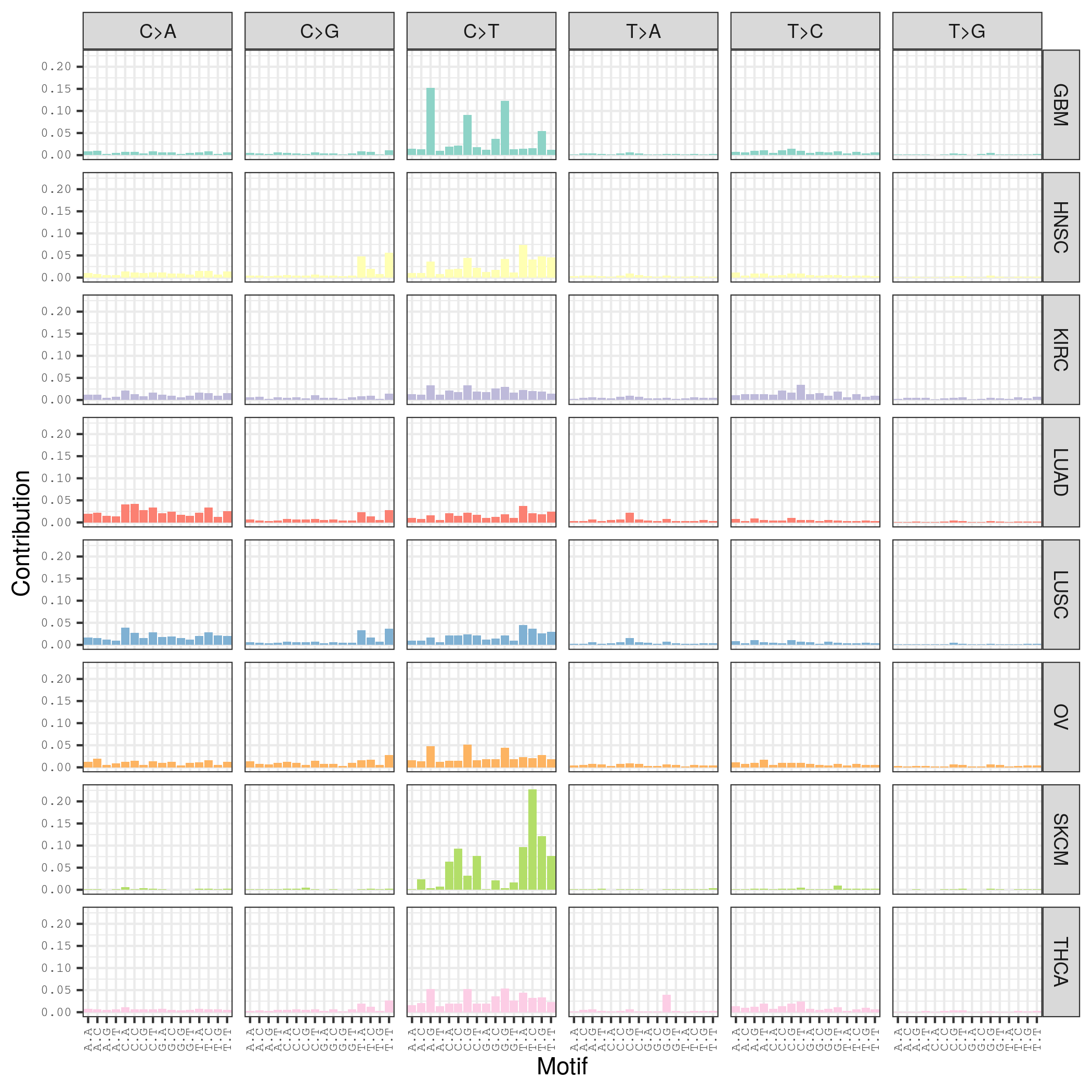Mutation spectrum over studies

### 4.3 Decomposition: Inferring Somatic Signatures

The somatic signatures can be estimated with each of the statistical methods implemented in the package. Here, we will use the NMF and PCA, and compare the results. Prior to the estimation, the number $$r$$ of signatures to obtain has to be fixed; in this example, the data will be decomposed into 5 signatures.

n_sigs = 5

sigs_nmf = identifySignatures(sca_mm, n_sigs, nmfDecomposition)


sigs_nmf

   MutationalSignatures:
Samples (8): GBM, HNSC, ..., SKCM, THCA
Signatures (5): S1, S2, S3, S4, S5
Motifs (96): CA A.A, CA A.C, ..., TG T.G, TG T.T

sigs_pca

   MutationalSignatures:
Samples (8): GBM, HNSC, ..., SKCM, THCA
Signatures (5): S1, S2, S3, S4, S5
Motifs (96): CA A.A, CA A.C, ..., TG T.G, TG T.T


The individual matrices can be further inspected through the accessors signatures, samples, observed and fitted.

### 4.4 Assessment: Number of Signatures

Up to now, we have performed the decomposition based on a known number $$r$$ of signatures. In many settings, prior biological knowledge or complementing experiments may allow to determine $$r$$ independently. If this is not the case, we can try to infer suitable values for $$r$$ from the data.

Using the assessNumberSignatures function, we can compute the residuals sum of squares (RSS) and the explained variance between the observed $$M$$ and fitted $$WH$$ mutational spectrum for different numbers of signatures. These measures are generally applicable to all kinds of decomposition methods, and can aid in choosing a likely number of signatures. The usage and arguments are analogous to the identifySignatures function.

n_sigs = 2:8

gof_nmf = assessNumberSignatures(sca_mm, n_sigs, nReplicates = 5)



The obtained statistics can further be visualized with the plotNumberSignatures. For each tested number of signatures, black crosses indicate the results of individual runs, while the red dot represents the average over all respective runs. Please note that having multiple runs is only relevant for randomly seeded decomposition methods, as the NMF in our example.

plotNumberSignatures(gof_nmf)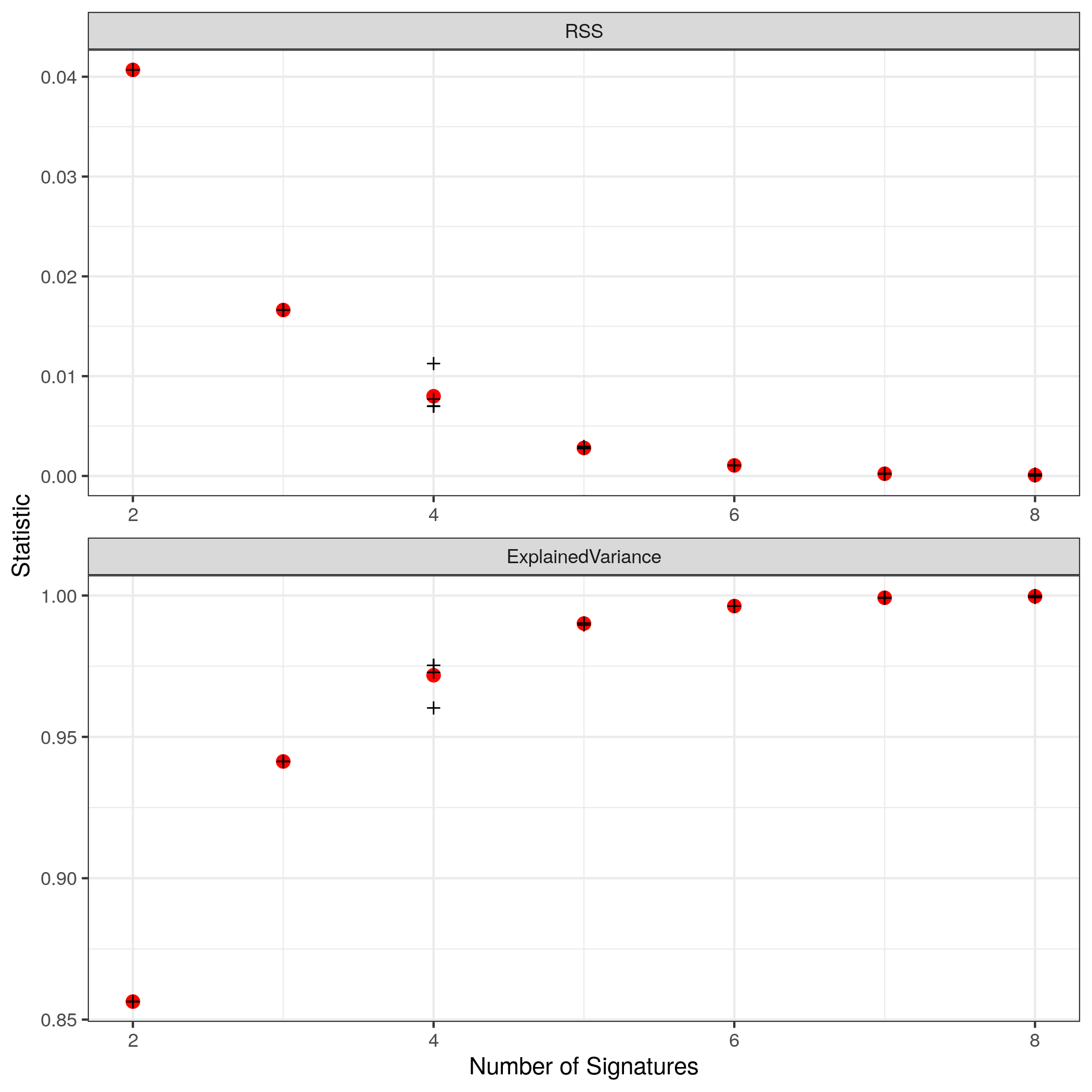Summary statistics for selecting the number of signatures in the NMF decomposition.

plotNumberSignatures(gof_pca)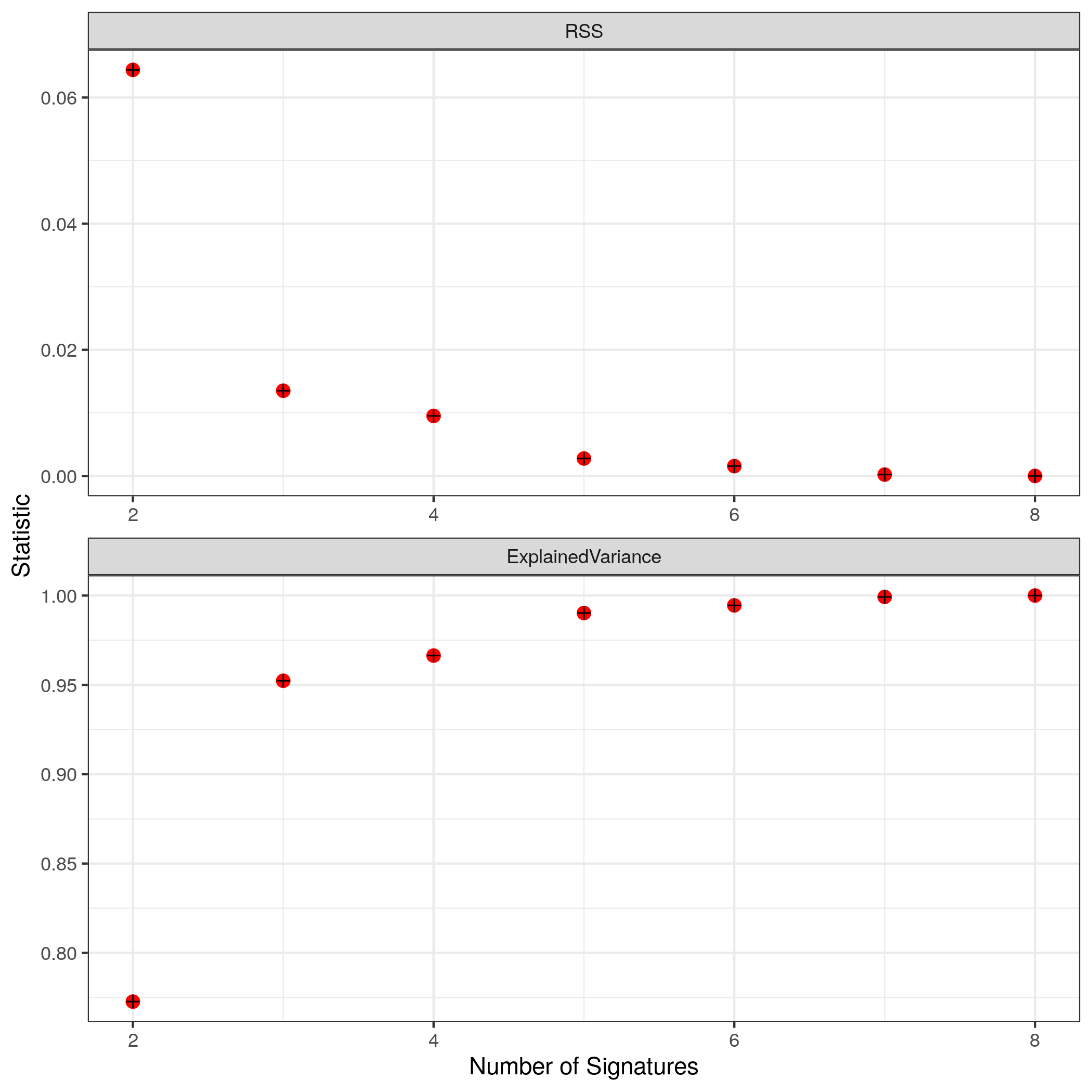Summary statistics for selecting the number of signatures in the PCA decomposition.

$$r$$ can then be chosen such that increasing the number of signatures does not yield a significantly better approximation of the data, i.e. that the RSS and the explained variance do not change sufficiently for more complex models. The first inflection point of the RSS curve has also been proposed as a measure for the number of features in this context . Judging from both statistics for our dataset, a total of 5 signatures seems to explain the characteristics of the observed mutational spectrum well. In practice, a combination of a statistical assessment paired with biological knowledge about the nature of the data will allow for the most reliable interpretation of the results.

### 4.5 Visualization: Exploration of Signatures and Samples

To explore the results for the TCGA data set, we will use the plotting functions. All figures are generated with the ggplot2 package, and thus, their properties and appearances can directly be modified, even at a later stage.

library(ggplot2)


Focusing on the results of the NMF first, the five somatic signatures (named S1 to S5) can be visualized either as a heatmap or as a barchart.

plotSignatureMap(sigs_nmf) + ggtitle("Somatic Signatures: NMF - Heatmap")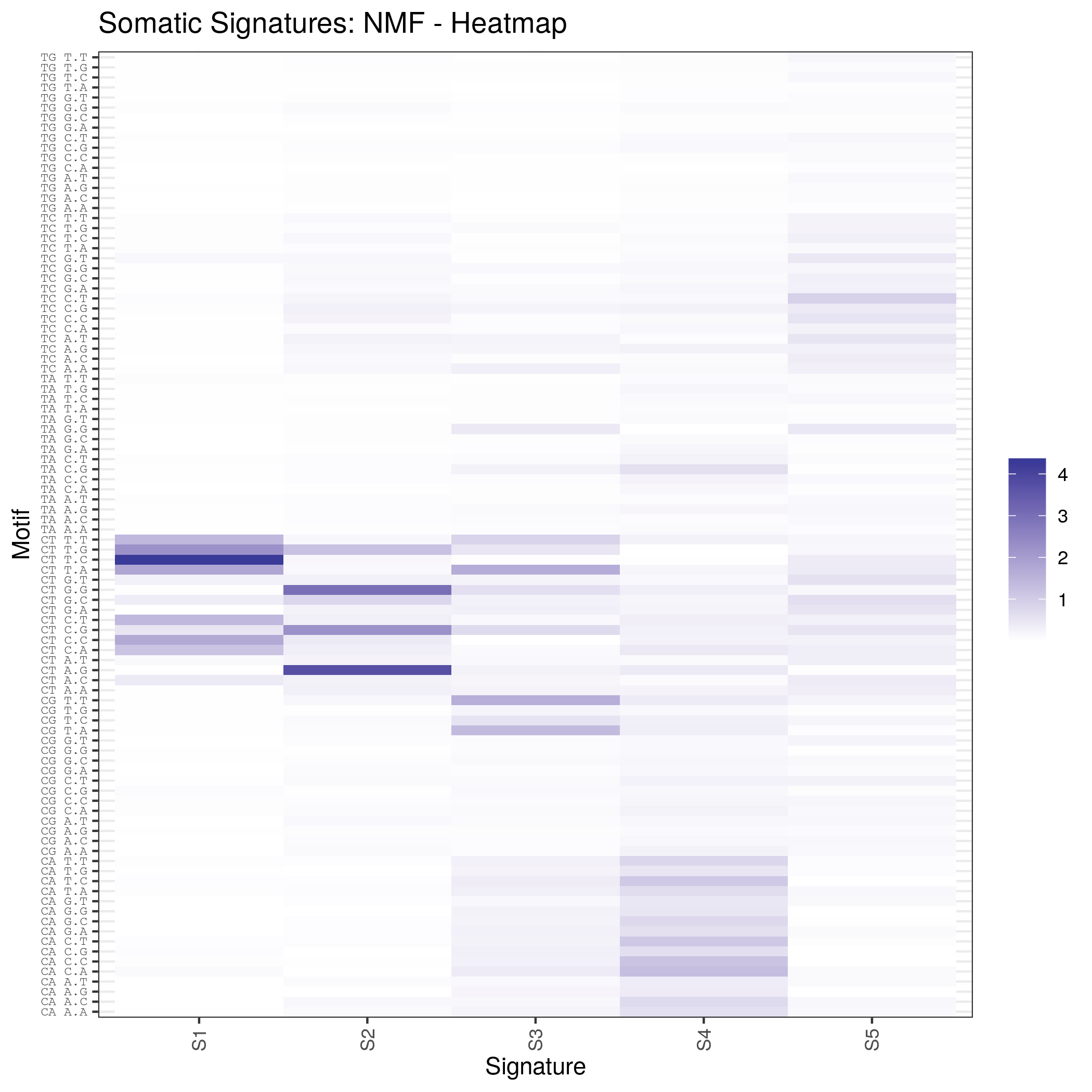Composition of somatic signatures estimated with the NMF, represented as a heatmap.

plotSignatures(sigs_nmf) + ggtitle("Somatic Signatures: NMF - Barchart")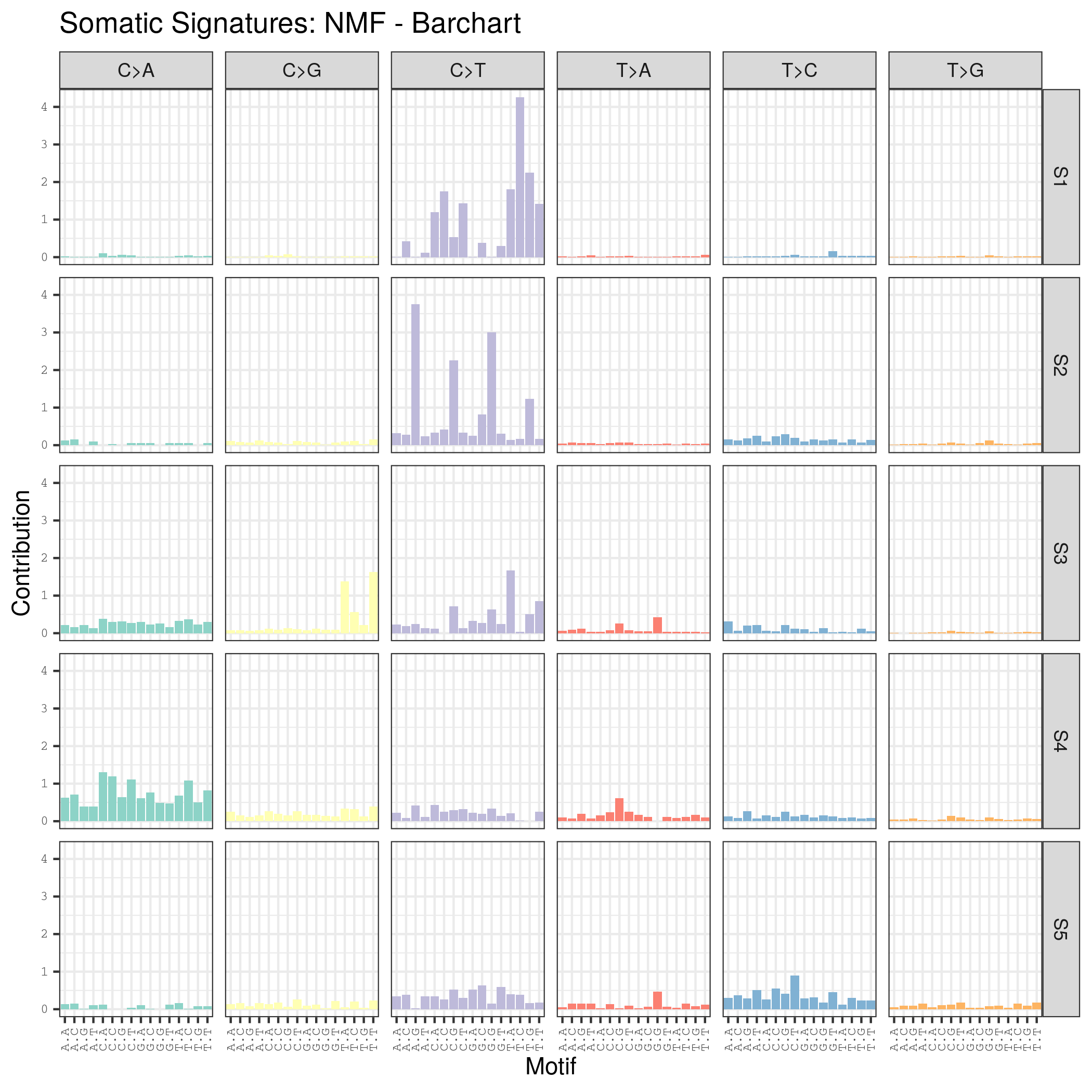Composition of somatic signatures estimated with the NMF, represented as a barchart.

plotObservedSpectrum(sigs_nmf)